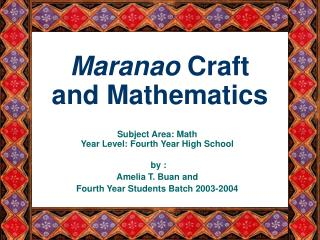DownloadDownload PresentationMaranao Craft and Mathematics

# Maranao Craft and Mathematics

Télécharger la présentation## Maranao Craft and Mathematics

- - - - - - - - - - - - - - - - - - - - - - - - - - - E N D - - - - - - - - - - - - - - - - - - - - - - - - - - -
##### Presentation Transcript

1. Subject Area: MathYear Level: Fourth Year High School by : Amelia T. Buan and Fourth Year Students Batch 2003-2004 Maranao Craft and Mathematics

2. Unit Summary This unit covers the topic on transformations in math. Geometiric transformation were discussed in graphing functions. In this unit, after students learned basic geometric transformation , they relate these to real life situations by filling out a journal and doing a research work on the geometric transformations used in Maranao crafts. Their work should also include famous Muslim mathematicians and their contributions to mathematics. This lesson is trying to connect mathematics to Maranao culture and historyto improve students appreciation on the local craft in Lanao del Norte specifically the Maranao Craft. The presentation of the students also includes their variation of the Maranao design they chose to present by applying the transformations they learned.

3. Unit Plan Unit Plan Title: MARANAO CRAFTS AND MATHEMATICS Curriculum-Framing Questions • How are Maranao designs created? Essential Question Unit Questions • How does mathematics relate to craft? • How do we make transformations in mathematics? • Who are the famous Muslim mathematicians? • How do Muslims use mathematics in their craft? • What geometric transformation are found in Maranao crafts? • How do we create designs using geometric transformation?

4. Student Samples Multimedia Presentations Landap and Mathematics Malong and Mathematics Design Using Arithmetic Sequence Journals

5. These designs were created using number patterns obtained from an arithmetic sequence Procedure 1. Select an infinite arithmetic sequence 2. Get the number pattern of the sequence 3. Choose an Angle of turn of the sequence, which follows spiral movement. 4. Prepare a graphing paper Plot the number pattern in a graphing paper 5. The cross-stitch set was used in constructing the different structures. The stitching process followed the procedure of plotting the number pattern in a graphing paper in procedure 4.

6. Infinite Arithmetic Progression with common difference of 1 and first term is 1, turning an angle of 45 degrees

7. Infinite Arithmetic Progression with common difference of 4 and first term is 1turning an angle of 90 degrees

8. Infinite Arithmetic Progression with common difference of 1 and first term is 1,turning an angle of 135 degrees

9. Infinite Arithmetic Progression with common difference of 2 and first term is 1,turning an angle of 45 degrees

10. Teacher Support Materials Graphing Calculator Activities Vertical and Horizontal Translation Exponential Function Exponential and Logarithmic Function Evaluation Tools Analytic Rubric for Math Journals Rubric for Multimedia Presentation